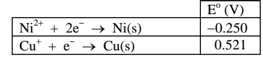# Problem: An electrochemical cell is constructed using the half-reactions given in the table below. A. What is the overall chemical equation for the  spontaneous redox reaction that would occur under standard conditions?     B. Which half-reaction is acting as the anode under standard conditions?    C. What is E° for this reaction?       D. What is n for this reaction? __________   E. Write the expression for Q for the reaction.   F. The cell was constructed with [Ni 2+] = 0.139 M and an unknown concentration of Cu + (aq). A potential of 0.604 V was measured for the cell under those conditions. What is the concentration of Cu+ (aq)?

###### FREE Expert Solution
86% (476 ratings)
###### Problem Details

An electrochemical cell is constructed using the half-reactions given in the table below.

A. What is the overall chemical equation for the  spontaneous redox reaction that would occur under standard conditions?

B. Which half-reaction is acting as the anode under standard conditions?

C. What is E° for this reaction?

D. What is n for this reaction? __________

E. Write the expression for Q for the reaction.

F. The cell was constructed with [Ni 2+] = 0.139 M and an unknown concentration of Cu + (aq). A potential of 0.604 V was measured for the cell under those conditions. What is the concentration of Cu+ (aq)?What scientific concept do you need to know in order to solve this problem?

Our tutors have indicated that to solve this problem you will need to apply the The Nernst Equation concept. You can view video lessons to learn The Nernst Equation. Or if you need more The Nernst Equation practice, you can also practice The Nernst Equation practice problems.

What is the difficulty of this problem?

Our tutors rated the difficulty ofAn electrochemical cell is constructed using the half-reacti...as high difficulty.

How long does this problem take to solve?

Our expert Chemistry tutor, Ellen took 11 minutes and 56 seconds to solve this problem. You can follow their steps in the video explanation above.

What professor is this problem relevant for?

Based on our data, we think this problem is relevant for Professor Allen's class at CSU.